Want to share your content on R-bloggers? click here if you have a blog, or here if you don't.

This is of course a rhetorical question! Because cyclists must stop when the light is red! … But … there is always that moment, on a bicycle, when you stop, and  then you say to yourself

the worst part is that the lights are badly regulated, and I know that the next one will also be red once I will reach it … whereas if I had passed, I would have had the next one, and who knows, maybe a green wave afterwards?

Not having wanted to try the experiment with my bike, I wanted to try using simulations, on my computer.

Let us assume that in my city, there are red lights every 250 m, and they go from red to green in 1 minute, and similarly from green to red. Then, I can use a simple loop, where I compute the time from home, each time I pass a light: either the light is green, I go through, and I do not wait; or the light is red, then I wait, until the end of the minute. Here is the code. First, I define the constant parameter, with lights every 250 m, light turning either red or green every 1 minute, and I assume a constant speed here, of 15/60 km per min (or 15km per hour).

```v = 15/60
d = .250
t = 1```

Suppose that the office is at 10 km from home. The code to compute the time is simply (I do add a random noise on the time it takes to reach the next light, I will get back to that later on)

```Dist = seq(0,10,by=.25)
Time1=rep(NA,length(D))
Time1==0
for(k in 2:length(Dist)){
noise=rnorm(1,sd=.05)
Time1[k]=Time1[k-1]+d/v+noise
if((floor(Time1[k])%%2==0)&(k<length(Dist))) Time1[k]=ceiling(Time1[k])
}```

I can now visualize myself on my bike

```T=seq(0,60,by=1/60)
colr = c("red","green")
plot(T,T*v,col="white")
for(k in 1:40){
points(T,rep(d*k,length(T)),cex=.3,pch=15,col=colr[rep(1:2,each=t*60)])
}
points(Time1,Dist,pch=19,cex=.5)```Here, it took me about 51 min to reach the office. And each time I have a red light, I stop and wait. Here is the distribution of the time it will take, as a function of my speed

```simul = function(v=.250,sd=.05){
Dist = seq(0,10,by=.25)
Time1=Time2=rep(NA,length(D))
Time1=Time2=0
for(k in 2:length(Dist)){
noise=rnorm(1,sd=sd)
Time1[k]=Time1[k-1]+d/v+noise
if((floor(Time1[k])%%2==0)&(k<length(Dist))) Time1[k]=ceiling(Time1[k])
}
max(Time1)
}
S=function(v=.250,sd=.05,n=1000){
Vectorize(function(x) simul(v=x,sd=sd))(rep(v,n))
}
vit = seq(.2,.3,length=101)
MS = Vectorize(function(x) S(v=x,sd=.05))(vit)
qMSsup = apply(MS,2,function(x) quantile(x,.95))
qMSinf = apply(MS,2,function(x) quantile(x,.05))
MSmed = apply(MS,2,function(x) quantile(x,.5))
MSmean = apply(MS,2,mean)
par(mfrow = c(1,1))
plot(vit,MSmed,type="l")
polygon(c(vit,rev(vit)),c(qMSinf,rev(qMSsup)),col="light blue",border=NA)
lines(vit,MSmed,lwd=2)```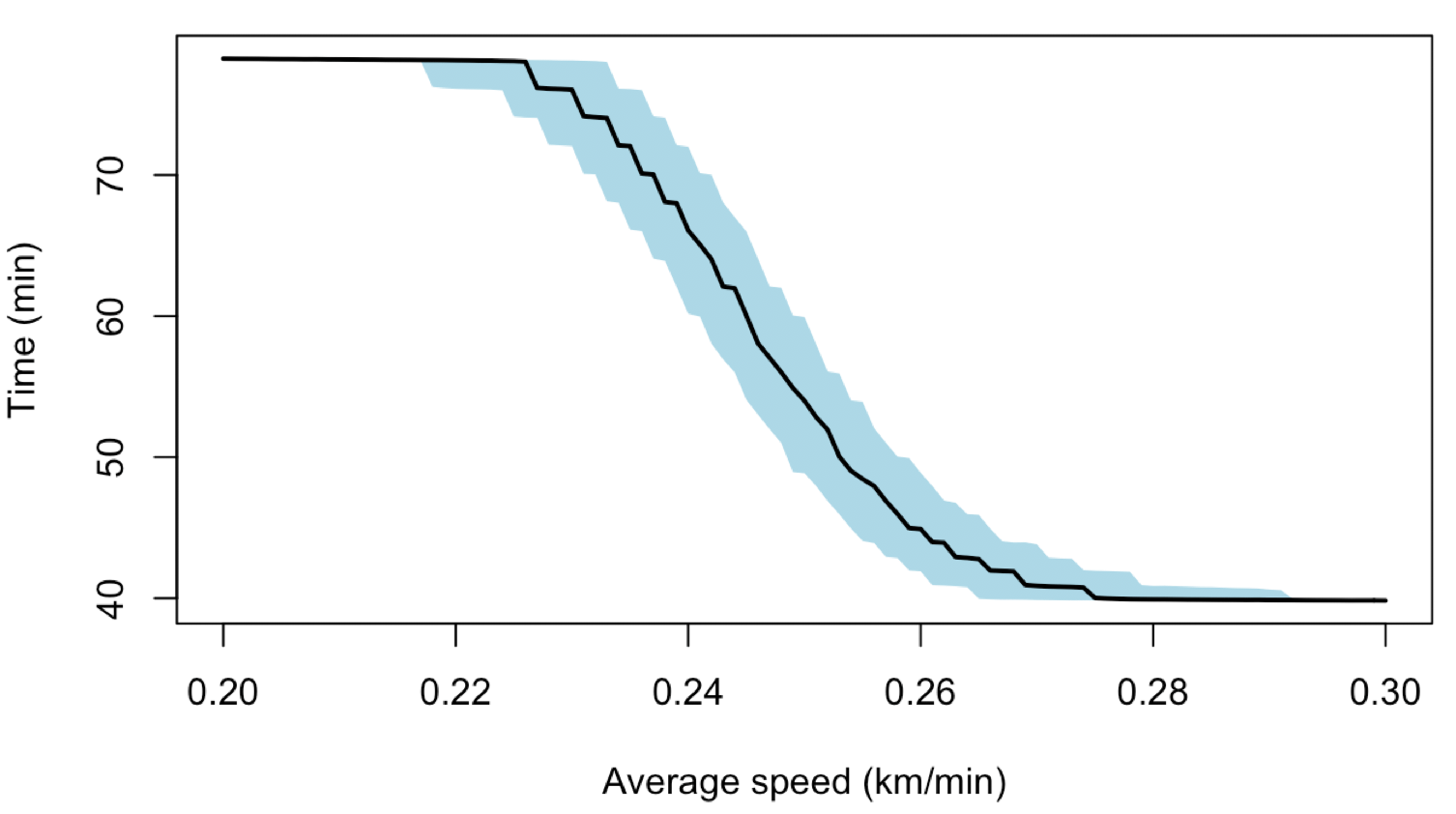Obviously, on average, the faster I cycle, the shorter the ride will be. Of course, 40 min is not a (real) lower bound : if I go much faster, it can be a rather quick rideNow, consider a simple rhetorical alternative. What if I decide to go through a red light (after checking that there is no danger), say, with 1 chance of out 20 ?

```simul_random = function(v=.250,sd=.05){
Dist = seq(0,10,by=.25)
Time1=rep(NA,length(D))
Time1=0
for(k in 2:length(Dist)){
noise=rnorm(1,sd=sd)
Time1[k]=Time1[k-1]+d/v+noise
red = sample(c(0,1),size=1,prob = c(.95,.05))
if((floor(Time1[k])%%2==0)&(k<length(Dist))&(red == 0)) Time1[k]=ceiling(Time1[k])
}
max(Time1)
}```

Here, 1 chance out of 20 could mean that on a 10 km ride, I wil always stop : first, I need a red light, and then 95% of the time, I stop. Here is the average distribution, with confidence bands

```S_random=function(v=.250,sd=.05,n=1000){
Vectorize(function(x) simul_random(v=x,sd=sd))(rep(v,n))
}
MS3 = Vectorize(function(x) S_random(v=x,sd=.05))(vit)
qMSsup3 = apply(MS3,2,function(x) quantile(x,.95))
qMSinf3 = apply(MS3,2,function(x) quantile(x,.05))
MSmed3 = apply(MS3,2,function(x) quantile(x,.5))```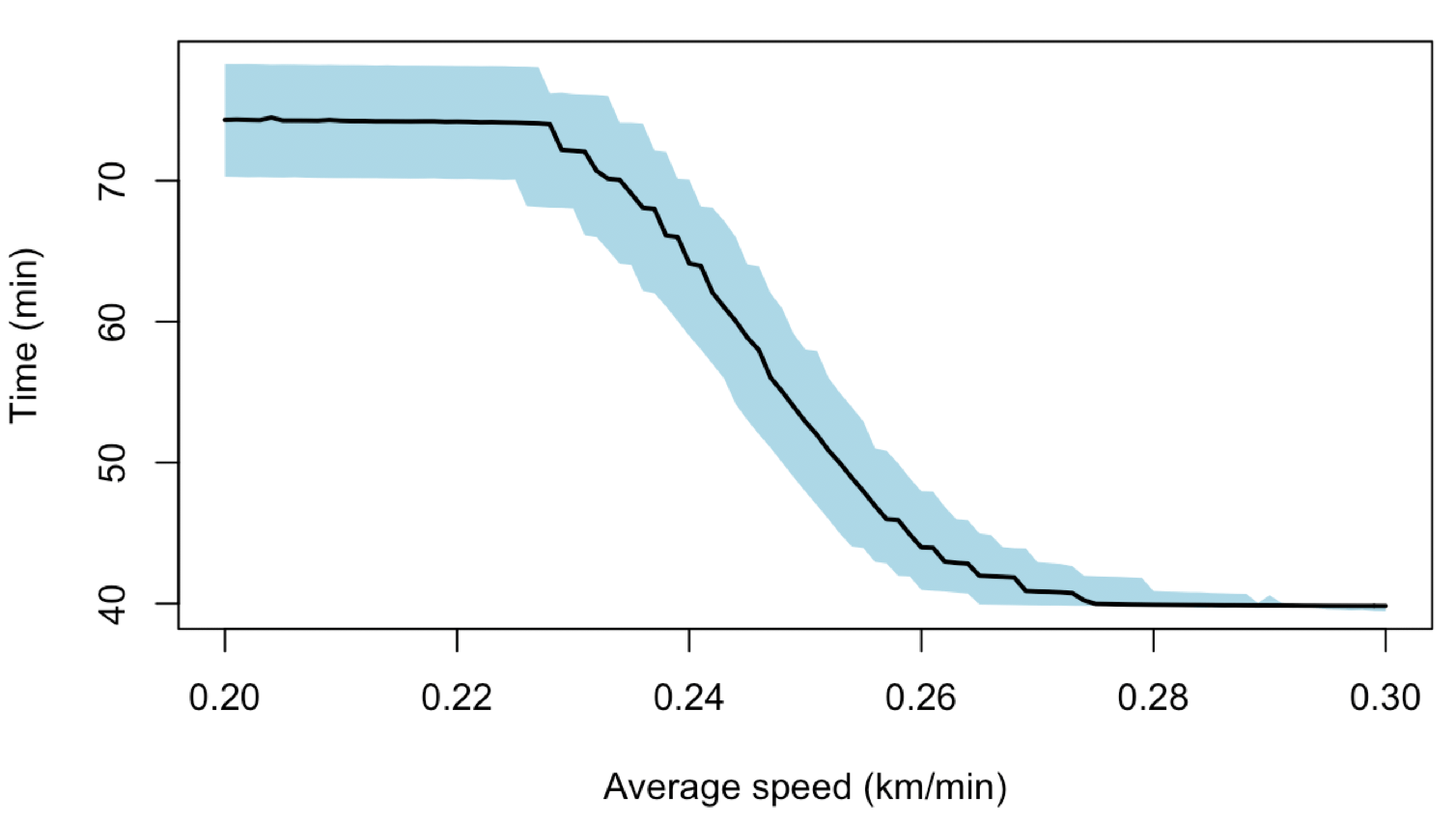It is possible to compare the two actually (on average times)

```plot(MSmed,MSmed3,type="l")
abline(a=0,b=1,col="red",lty=2)
plot(MSmed,(MSmed-MSmed3)/MSmed*100,type="l")```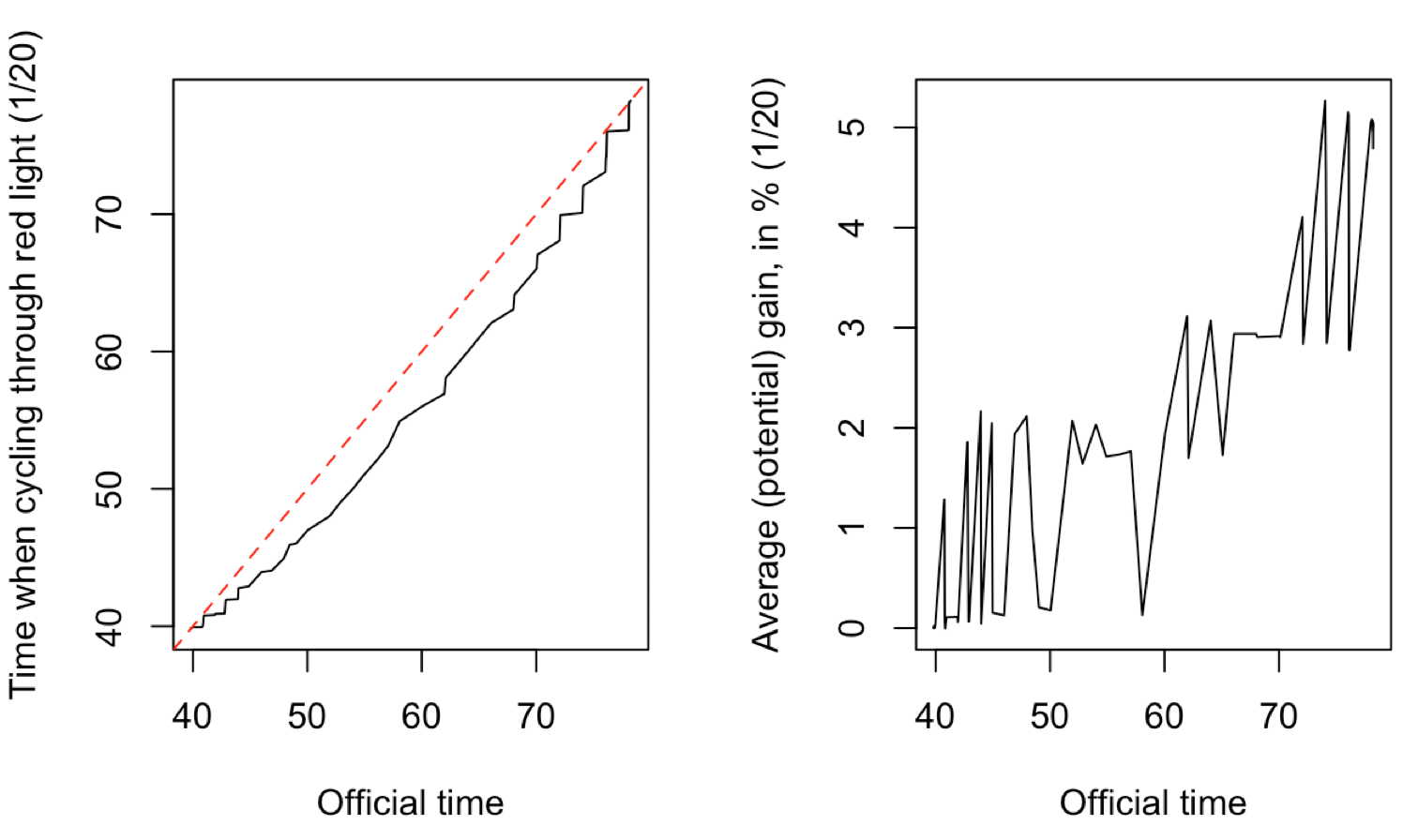which means that, somehow I can save some time, maybe from 3% to 5% if I do not cycle to fast, otherwise probably less than 2%. I would not claim, here, that it is worth it.

Consider another rhetorical alternative. What if I decide to go through the red light only if it is during the very first second ?

```simul_1sec = function(v=.250,sd=.05){
Dist = seq(0,10,by=.25)
Time1=rep(NA,length(D))
Time1=0
for(k in 2:length(Dist)){
noise=rnorm(1,sd=sd)
Time1[k]=Time1[k-1]+d/v+noise
if((floor(Time1[k])%%2==0)&(k<length(Dist))&(Time1[k]-floor(Time1[k])>1/60)) Time1[k]=ceiling(Time1[k])
}
max(Time1)
}```

Here is the time it takes to go to the officeand here is the potential gain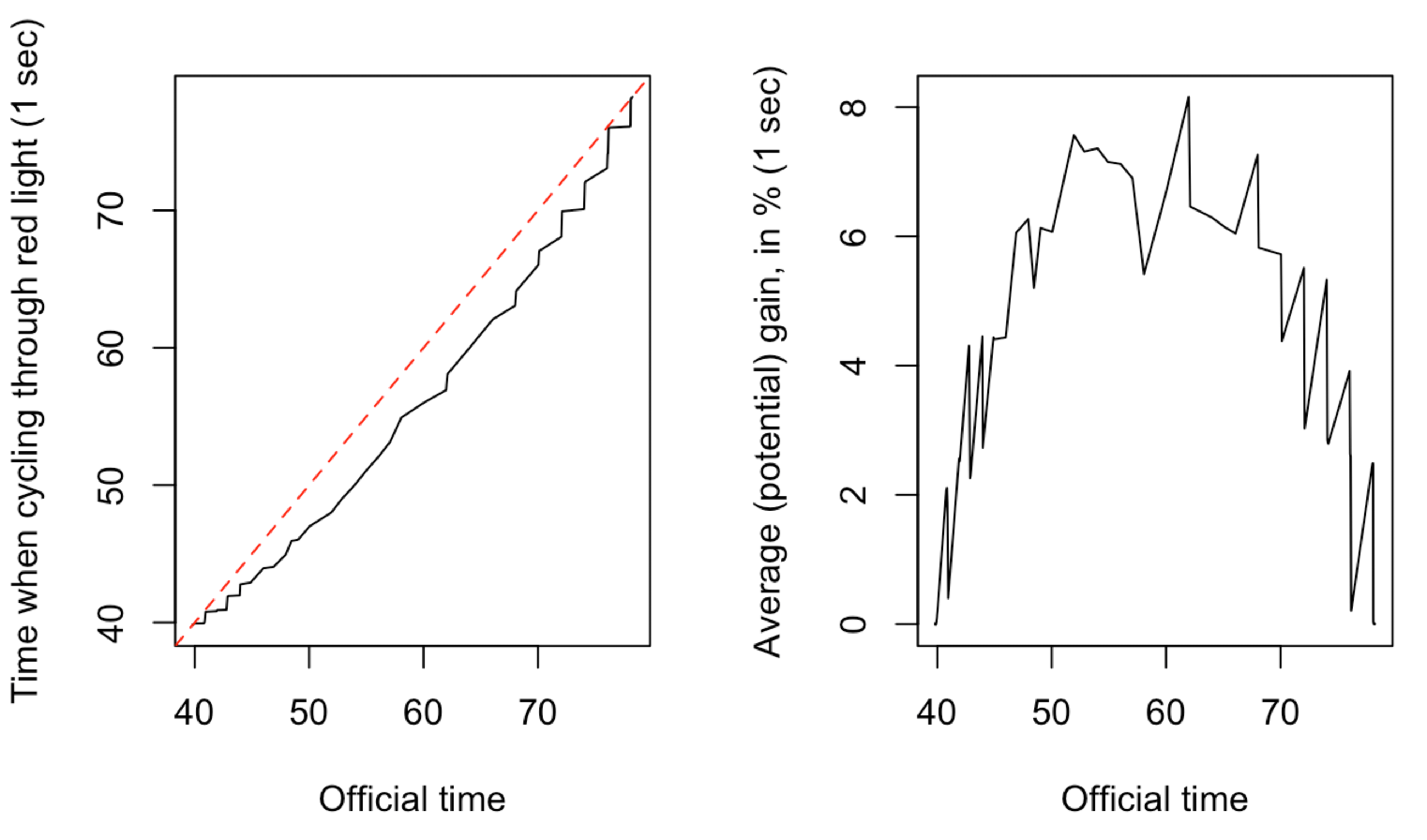We can now clearly see the impact of those nonlinearities : with my average 15 km per hour speed, I can save 8% of the time if I go through red during the very first second. Of course, I will never do such a think, but mathematically speaking, it is stricking. Here is the gain for the first 2 seconds (out of a full minute)so the gain can be about 12%. And now, what if we remove the noise – or more precisely, what if the standard deviation become 0.001 instead of 0.05. Here is the first distribution of the time (when I stop at each red light, and wait)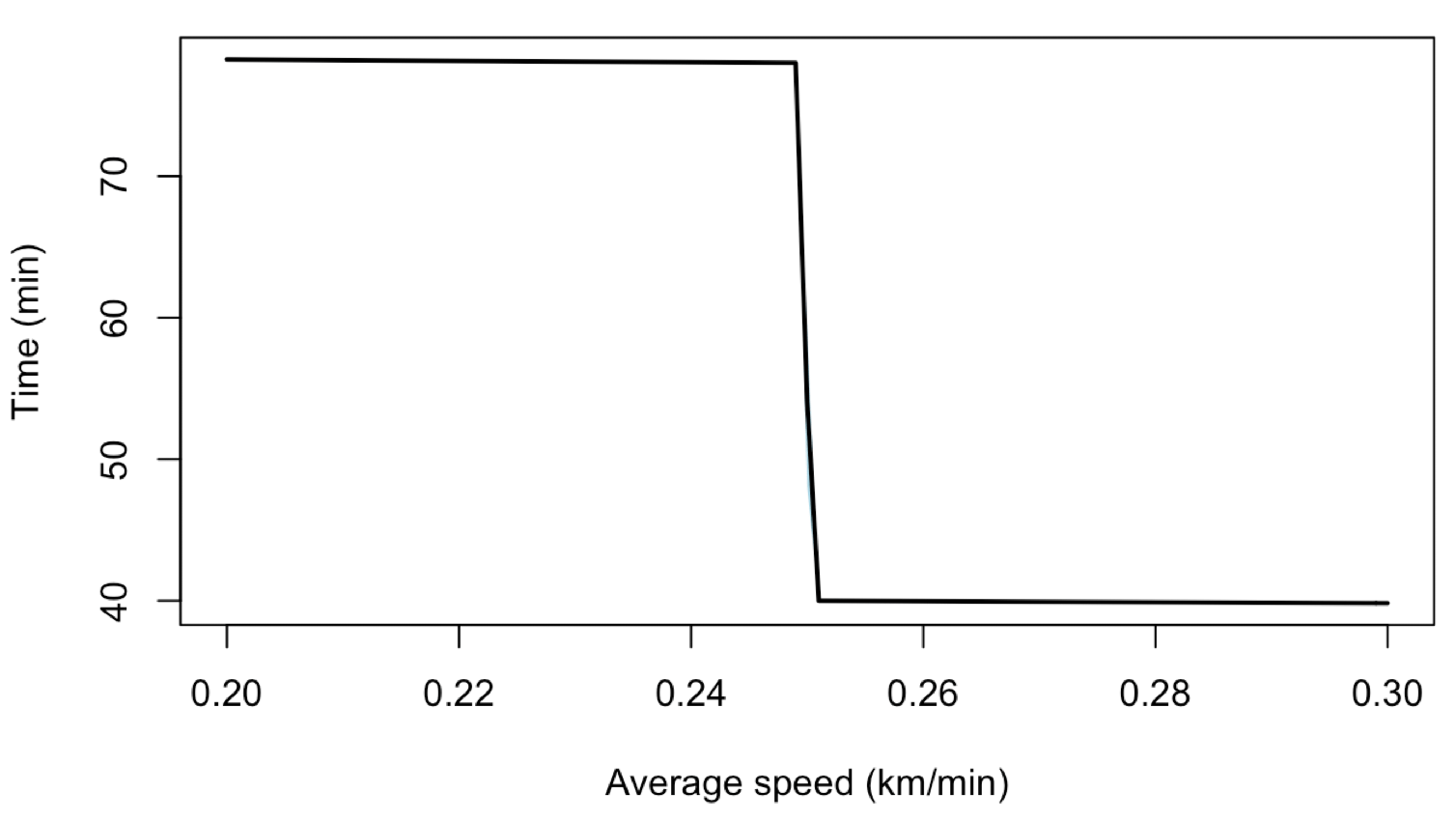If I do not go fast enough, I will stop at almost each light, and wait until the end of the minute: going at 20 km per hour or 24 km per hour is exactly the same. But if I can go slightly faster than 25km per hour, that is awesome, and I have my green wave. Now, her is the graph I get when I cycle through the red light only in the very first secondThis is now the gainI can save up to 40% of the time, and more realistically, my 55 min ride could now be a 40 min ride. Which is substantial.

But on that one, observe that another strategy is also possible : what if I do not cycle through red light, but I simply cycle +1% faster ?

```simul_faster = function(v=.250,sd=.05){
Dist = seq(0,10,by=.25)
Time1=rep(NA,length(D))
Time1=0
for(k in 2:length(Dist)){
noise=rnorm(1,sd=sd)
Time1[k]=Time1[k-1]+d/(v*1.01)+noise
if((floor(Time1[k])%%2==0)&(k<length(Dist))&(Time1[k]-floor(Time1[k])>1/60)) Time1[k]=ceiling(Time1[k])
}
max(Time1)
}```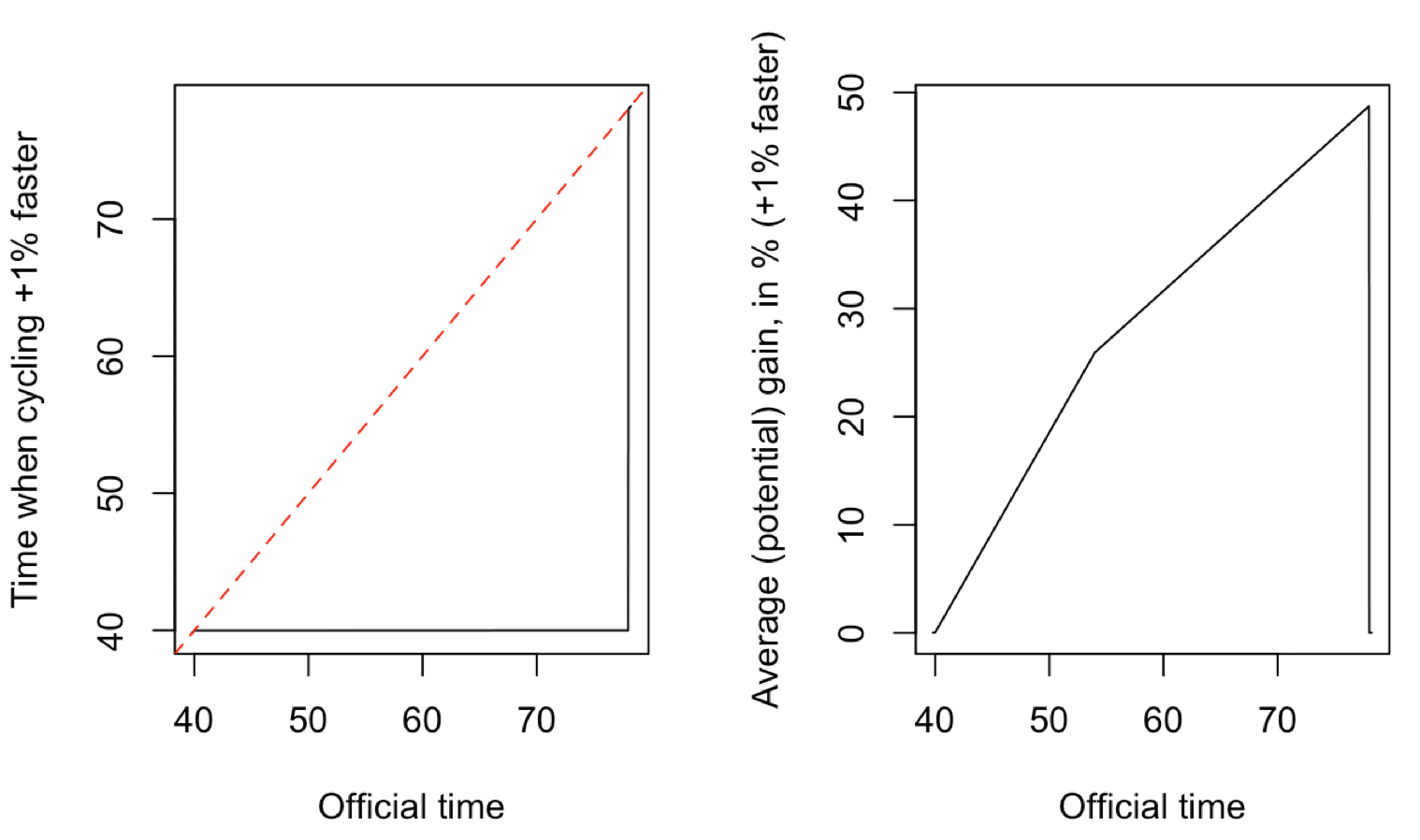We have a similar result here, since riding 1% faster can actually help me save up to 50% of my time ! and (more realistically) if I ride 1% faster with a large random noise in the time between two light, I get almost the same as previously (when passing through the red light)Again, my point here is not that we should cycle through a red light, of course not. But there may be accumulation of (small) nonlinear effects that might have a major impact at the end. And I believe that the best way to avoid this is to offer a green wave to cyclists, assuming that they ride at a reasonnable speed…